# Step 3: Creating a Design Using Discrete Components - 2020.2 English

Document ID
UG1498
Release Date
2021-01-22
Version
2020.2 English
In this step you will see how System Generator can be used to build a design using discrete components to realize a very efficient hardware design.
1. At the command prompt, type ```open Lab1_3.slx```.

This opens the Simulink design shown in the following figure. This design is similar to the one in the previous two steps. However, this time the filter is designed with discrete components and is only partially complete. As part of this step, you will complete this design and learn how to add and configure discrete parts.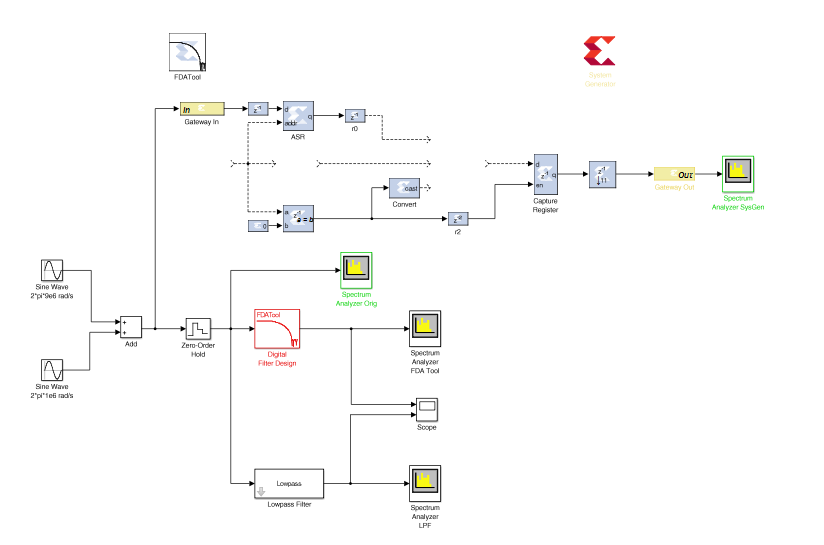This discrete filter operates in this way:

• Samples arrive through port In and after a delay stored in a shift register (instance ASR).
• A ROM is required for the filter coefficients.
• A counter is required to select both the data and coefficient samples for calculation.
• A multiply accumulate unit is required to perform the calculations.
• The final down-sample unit selects an output every nth cycle.

Start by adding the discrete components to the design.

2. Click the Library Browser button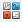in the Simulink toolbar to open the Simulink Library Browser.
1. Expand the Xilinx Blockset menu.
2. As shown in the following figure, select the Control Logic section, then right-click Counter to add this component to the design.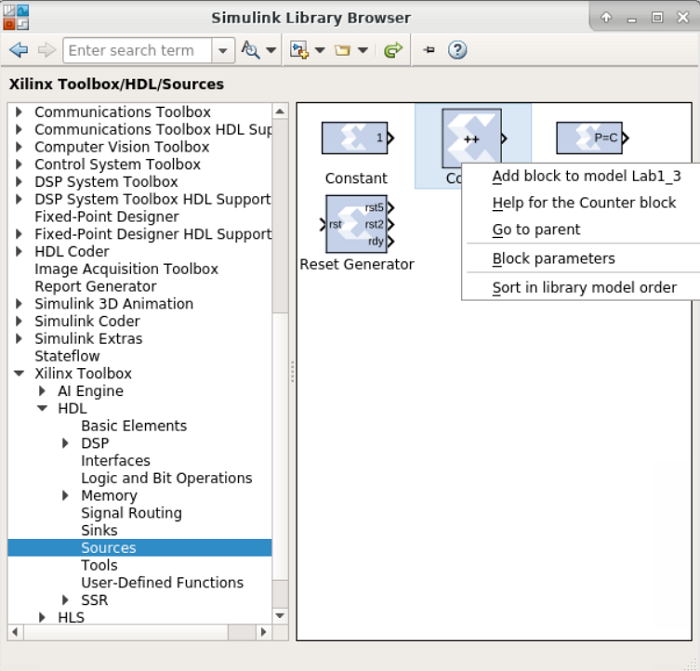3. Select the Memory section (shown at the bottom left in the figure above) and add a ROM to the design.
4. Finally, select the DSP section and add a DSP48 Macro 1.0 to the design.
3. Connect the three new instances to the rest of the design as shown in the following figure: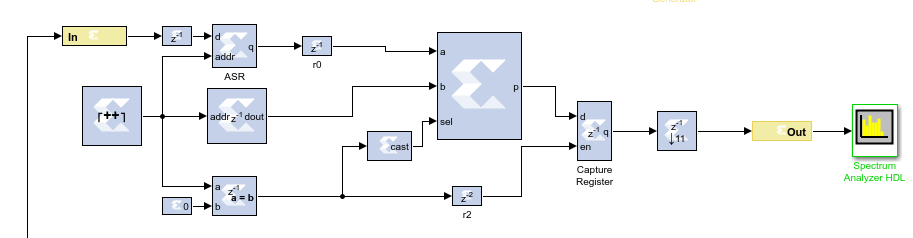You will now configure the instances to correctly filter the data.

4. Double-click the FDATool instance and select Filter Coefficients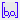from the toolbar to review the filter specifications.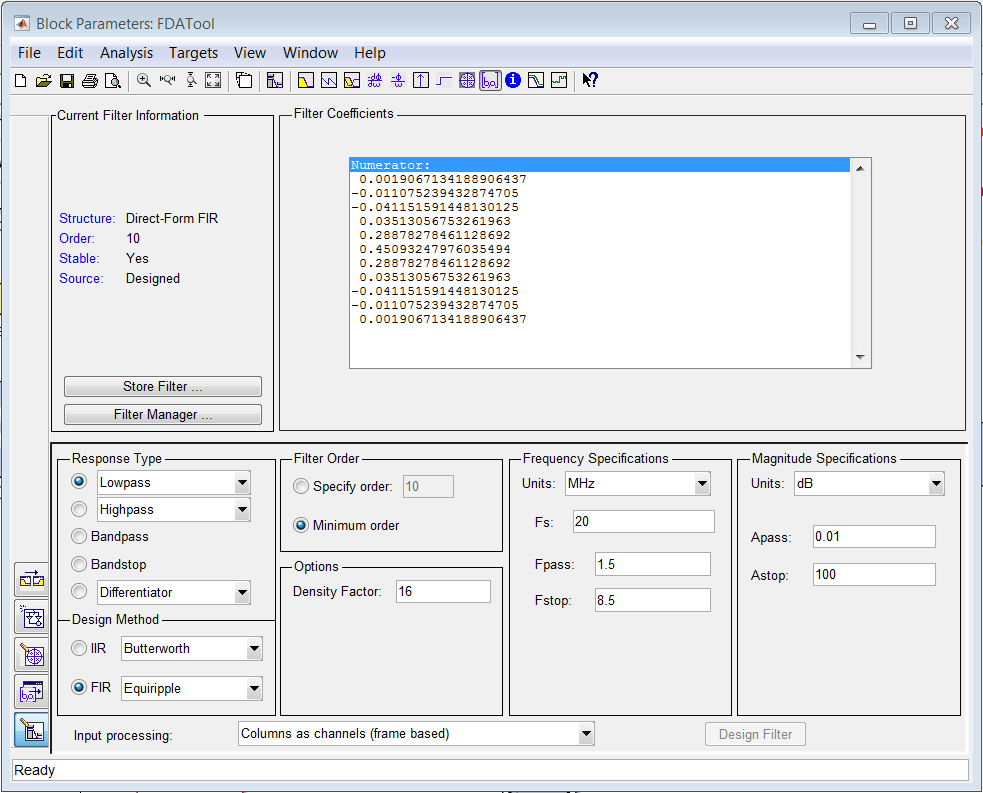This shows the same specifications as the previous steps in Lab 1 and confirms there are 11 coefficients. You can also confirm, by double-clicking on the input Gateway In that the input sample rate is once again 20 MHz (Sample period = 1/20e6). With this information, you can now configure the discrete components.

5. Close the FDATool Properties Editor.
6. Double-click the Counter instance to open the Properties Editor.
1. For the Counter type, select Count limited and enter this value for Count to value: `length(xlfda_numerator('FDATool'))-1`

This will ensure the counter counts from 0 to 10 (11 coefficient and data addresses).

2. For Output type, leave default value at Unsigned and in Number of Bits enter the value 4. Only 4 binary address bits are required to count to 11.
3. For the Explicit period, enter the value 1/(11*20e6) to ensure the sample period is 11 times the input data rate. The filter must perform 11 calculations for each input sample.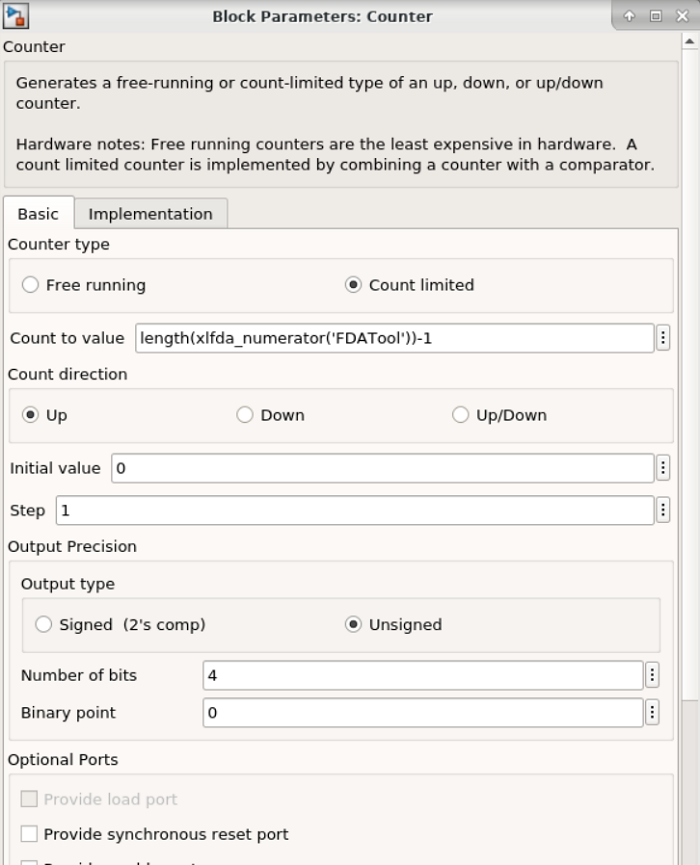4. Click OK to exit the Properties Editor.
7. Double-click the ROM instance to open the Properties Editor.
1. For the Depth, enter the value `length(xlfda_numerator('FDATool'))`. This will ensure the ROM has 11 elements.
2. For the Initial value vector, enter `xlfda_numerator('FDATool')`. The coefficient values will be provided by the FDATool instance.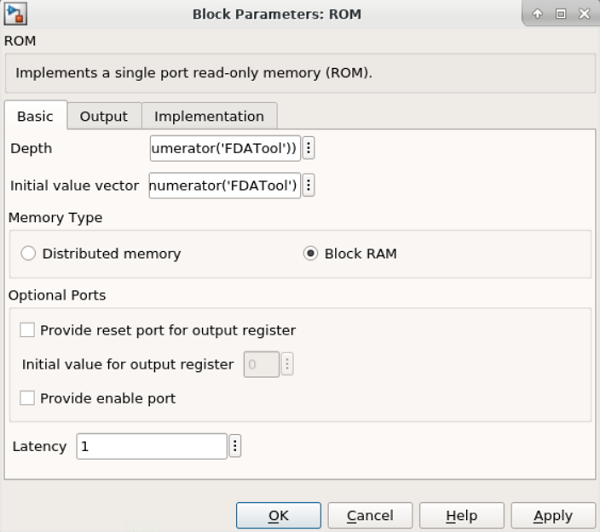3. Click OK to exit the Properties Editor.
8. Double-click the DSP48 Macro 1.0 instance to open the Properties Editor.
1. In the Instructions tab, replace the existing Instructions with `A*B+P` and then add `A*B`. When the `sel` input is false the DSP will multiply and accumulate. When the `sel` input is true the DSP will simply multiply.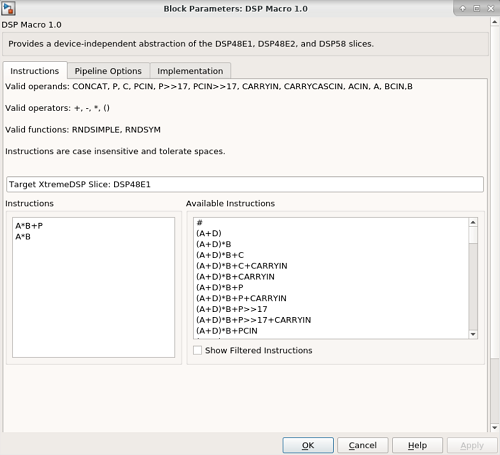2. In the Pipeline Options tab, use the Pipeline Options drop-down menu to select By_Tier.
3. Select Tier 3 and Tier 5. This will ensure registers are used at the inputs to A and B and between the multiply and accumulate operations.